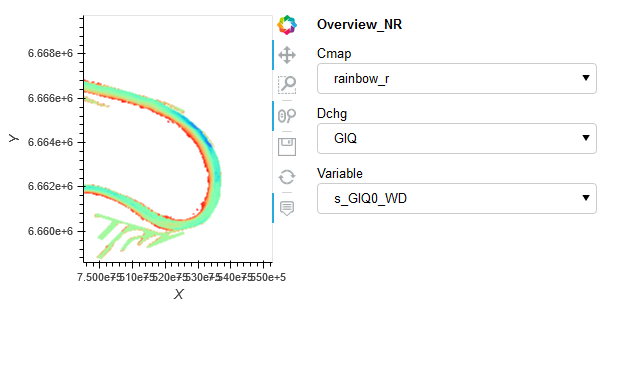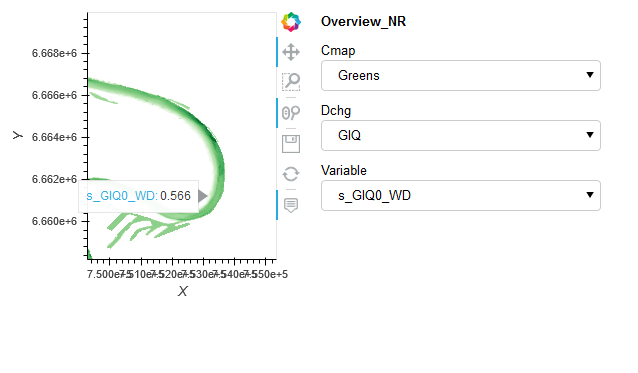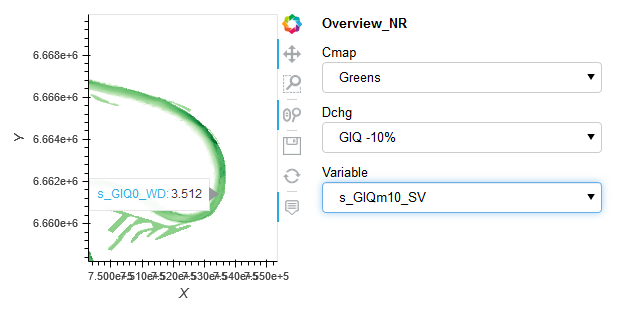# Using @param.depends in a parameterized Class

Hi everybody,
I am developing a Panel based Dashboard where I can change the dynamic Map. The different rasterized Trimesh maps are stored in a Dict called ‘Trimesh_dict’. I defined 3 Parameters: Two “ParamSelects” are linked to change the Dropdown of the parameter selection which set/change the key of the dictionairy.
Additionally the cmap is changed by Parameter Selection as well. Sometimes changing the cmap works but the selection of the Parameter ‘Variable’ do not affect the Dictionairys key.
I am sorry that I did not manage to set up a miminal Example. I think I did some fundamental mistake using the param Class. Maybe you can give me some advise.
I am using the hv.extension(‘bokeh’) and pn.extension()

The overall question I am dealing with is why the of method view() does not change when I select another ‘Variable’ even though I set watch=True.

I think I am missing the init method, but I am a little confused, when I need it and how to use it…

cheers Fabian

``````df_masked=pd.concat([df['X'], df['Y'], s_GlQ0_WD, s_GlQp5_WD, s_GlQm10_WD, s_GlQm20_WD, s_GlQm30_WD,
s_GlQ0_SV, s_GlQp5_SV, s_GlQm10_SV, s_GlQm20_SV, s_GlQm30_SV,
s_GlQ0_BF, s_GlQp5_BF, s_GlQm10_BF, s_GlQm20_BF, s_GlQm30_BF], axis=1)

vdims = ['s_GlQ0_WD', 's_GlQp5_WD', 's_GlQm10_WD', 's_GlQm20_WD', 's_GlQm30_WD', 's_GlQ0_SV', 's_GlQp5_SV', 's_GlQm10_SV', 's_GlQm20_SV', 's_GlQm30_SV', 's_GlQ0_BF', 's_GlQp5_BF', 's_GlQm10_BF', 's_GlQm20_BF', 's_GlQm30_BF']
cmap_sel={'viridis':'viridis', 'rainbow_r':'rainbow_r', 'cwr_r':'cwr_r','BrBG':'BrBG', 'coolwarm_r':'coolwarm_r'}
cmap_sel_list=['viridis','rainbow_r','Greens', 'Blues', 'cwr_r', 'BrBG', 'coolwarm_r']

tooltips_r= {}
trimesh_dict={}

for vdim in vdims:
points = gv.Points(df_masked, crs=crs.epsg(25832), kdims=['X', 'Y'], vdims = vdim)
tooltips_r[vdim] = [(vdim, '@image')]
d = gv.Dataset(gv.operation.project_points(points))
trimesh_dict[vdim] = rasterize(hv.TriMesh((element, d)), aggregator=ds.mean(vdim), precompute=True, dynamic=True).opts(
cmap=cmap_sel)
class Overview_NR(param.Parameterized):
q_select_opts = ['GlQ', 'GlQ +5%', 'GlQ -10%', 'GlQ -20%', 'GlQ -30%']
cmap = param.ObjectSelector(default='Greens', objects=cmap_sel_list)
dchg = param.ObjectSelector(default='GlQ', objects=q_select_opts)
variable = param.ObjectSelector(default = 's_GlQ0_WD', objects=['s_GlQ0_WD', 's_GlQ0_SV', 's_GlQ0_BF'])
_variables = {'GlQ': ['s_GlQ0_WD', 's_GlQ0_SV', 's_GlQ0_BF'],
'GlQ +5%':['s_GlQp5_WD', 's_GlQp5_SV', 's_GlQp5_BF'],
'GlQ -10%':['s_GlQm10_WD', 's_GlQm10_SV', 's_GlQm10_BF'],
'GlQ -20%':['s_GlQm20_WD', 's_GlQm20_SV', 's_GlQm20_BF'],
'GlQ -30%':['s_GlQm30_WD', 's_GlQm30_SV', 's_GlQm30_BF']}

@param.depends('dchg', watch=True)
def _update_Variablen(self):
variables = self._variables[self.dchg]
self.param['variable'].objects = variables
self.variable = variables

#the method inkluding param decorator shoud return the  requested DynamincMap
@param.depends('variable', 'cmap', watch=True)
def view(self):
hover=HoverTool(tooltips=Tooltips_R[self.variable])
return Trimesh_dict[self.variable].opts(cmap=self.cmap, tools=[hover])

ov_nr = Overview_NR()
layout = pn.Row(ov_nr.view(), ov_nr.param)

server = pn.serve(layout, start=False, show=True)``````

Can you show the code where you use the class and run the panel?

A pedantic side-note: Your code becomes easier to read if you don’t use caps for variables. Conventions are to use all lowercase for variables and functions, CamelCase for class names, and FULL_CAPS for constants.

I think you are trying to do something like this:

``````import panel as pn
import param

pn.extension()

class Overview(param.Parameterized):

OPTIONS = {'a': ['a1', 'a2'], 'b': ['b1', 'b2']}
selector_1 = param.ObjectSelector(default='a', objects=['a', 'b'])
selector_2 = param.ObjectSelector(default='a1', objects=['a1', 'a2'])

@param.depends('selector_1', watch=True)
def changes(self):
self.param['selector_2'].objects = self.OPTIONS[self.selector_1]

@param.depends('selector_2')
def view(self):
return self.param

model = Overview()
pn.serve(pn.panel(model.view))
``````

Does it do what you want? And if so, what is wrong with the following simpler solution?

``````import panel as pn
import param

pn.extension()

class Overview(param.Parameterized):

OPTIONS = {'a': ['a1', 'a2'], 'b': ['b1', 'b2']}
selector_1 = param.ObjectSelector(default='a', objects=['a', 'b'])
selector_2 = param.ObjectSelector(default='a1', objects=['a1', 'a2'])

@param.depends('selector_1')
def view(self):
self.param['selector_2'].objects = self.OPTIONS[self.selector_1]
return self.param

model = Overview()
pn.serve(pn.panel(model.view))``````

Hi @Leonidas,
I edited the code a little bit. I hope you feel confortable with my lowercase variables.

As you see at the screenshots below the colormap selection works after zooming. The two images with hover show that the change of ‘variable’ does not change the displayed data.Showing the full code helps! I think the problem might be that you use `ov_nr.view()` but can you try `ov_nr.view`. How it all works under the hood, I am not exactly sure, but I think you want to give panel a callback function such that it can be called when needed, in stead of only when you define your panel.

You only need an init if you want to initialize more things than the param constructor is initializing :). That could be done like so:

``````def __init__(self, **params):
super().__init__(**params)
3 Likes

Thank you, without the braces it works like I am expecting it.1 Like

Hi @sunny

If possible feel free to share the working code. That can be helpful for the community. The plot looks quite interesting.

Thanks.

Thats the working code I actually use. Due to the missing Data it’s not a working example.

``````df_masked=pd.concat([df['X'], df['Y'], s_GlQ0_WD, s_GlQp5_WD, s_GlQm10_WD, s_GlQm20_WD, s_GlQm30_WD,
s_GlQ0_SV, s_GlQp5_SV, s_GlQm10_SV, s_GlQm20_SV, s_GlQm30_SV,
s_GlQ0_BF, s_GlQp5_BF, s_GlQm10_BF, s_GlQm20_BF, s_GlQm30_BF], axis=1)

vdims = ['s_GlQ0_WD', 's_GlQp5_WD', 's_GlQm10_WD', 's_GlQm20_WD', 's_GlQm30_WD', 's_GlQ0_SV', 's_GlQp5_SV', 's_GlQm10_SV', 's_GlQm20_SV', 's_GlQm30_SV', 's_GlQ0_BF', 's_GlQp5_BF', 's_GlQm10_BF', 's_GlQm20_BF', 's_GlQm30_BF']
cmap_sel={'viridis':'viridis', 'rainbow_r':'rainbow_r', 'cwr_r':'cwr_r','BrBG':'BrBG', 'coolwarm_r':'coolwarm_r'}
cmap_sel_list=['viridis','rainbow_r','Greens', 'Blues', 'cwr_r', 'BrBG', 'coolwarm_r']

tooltips_r= {}
trimesh_dict={}

for vdim in vdims:
points = gv.Points(df_masked, crs=crs.epsg(25832), kdims=['X', 'Y'], vdims = vdim)
tooltips_r[vdim] = [(vdim, '@image')]
d = gv.Dataset(gv.operation.project_points(points))
trimesh_dict[vdim] = rasterize(hv.TriMesh((element, d)), aggregator=ds.mean(vdim), precompute=True, dynamic=True).opts(
cmap=cmap_sel)
class Overview_NR(param.Parameterized):
q_select_opts = ['GlQ', 'GlQ +5%', 'GlQ -10%', 'GlQ -20%', 'GlQ -30%']
cmap = param.ObjectSelector(default='Greens', objects=cmap_sel_list)
dchg = param.ObjectSelector(default='GlQ', objects=q_select_opts)
variable = param.ObjectSelector(default = 's_GlQ0_WD', objects=['s_GlQ0_WD', 's_GlQ0_SV', 's_GlQ0_BF'])
_variables = {'GlQ': ['s_GlQ0_WD', 's_GlQ0_SV', 's_GlQ0_BF'],
'GlQ +5%':['s_GlQp5_WD', 's_GlQp5_SV', 's_GlQp5_BF'],
'GlQ -10%':['s_GlQm10_WD', 's_GlQm10_SV', 's_GlQm10_BF'],
'GlQ -20%':['s_GlQm20_WD', 's_GlQm20_SV', 's_GlQm20_BF'],
'GlQ -30%':['s_GlQm30_WD', 's_GlQm30_SV', 's_GlQm30_BF']}

@param.depends('dchg', watch=True)
def _update_Variablen(self):
variables = self._variables[self.dchg]
self.param['variable'].objects = variables
self.variable = variables

#the method inkluding param decorator shoud return the  requested DynamincMap
@param.depends('variable', 'cmap', watch=True)
def view(self):
hover=HoverTool(tooltips=tooltips_r[self.variable])
return trimesh_dict[self.variable].opts(cmap=self.cmap, tools=[hover])

ov_nr = Overview_NR()
layout = pn.Row(ov_nr.view, ov_nr.param)

server = pn.serve(layout, start=False, show=True)
``````
1 Like